Got Feedback?

Found a bug? Have a suggestion? Fill the form below and we'll take a look!

X

# Pythagorean Theorem Calculator

Pythagorean Theorem calculator is used to find out the unknown side of a right angle triangle if two sides are known. It provides the accurate result of desired data and have ability to solve any real life problem which is directly or indirectly related to the right angle triangle. Let, the known sides of right angle triangle are 4cm and 5cm, for finding third side (hypotenuse). We have to consider Pythagorean Theorem by substituting the values in the formula.

$$a^2 + b^2 = c^2$$

$$\sqrt{}$$

$$\sqrt{}$$

## What is Pythagoras Theorem?

Pythagoras Theorem explains the relationship between base, perpendicular and hypotenuse of a right angled triangle (⊿).
Pythagoras Theorem states that in a right angled triangle, the square of the hypotenuse is equal to the sum of the squares of the remaining two sides. The sides of the right angle have been named as perpendicular, base and hypotenuse. The hypotenuse is the largest side and it is opposite to the right angle. (i-e. 90°)

## Pythagoras Theorem proof

It is the most important concept not only in mathematic but also it has a great influence on entire science.
In a right angle triangle hypotenuse has a special relation with rest of the other two sides.$$(Hyp)^2 = Sum of squares of other two sides$$

According to given figure, its exact pattern is like this, $$(Hyp)^2 = (Perpendicular)^2 + (Base)^2$$
As Hyp = c, Perpendicular = a, and Base = b. So, $$(c)^2 = (a)^2 + (b)^2$$
It is pretty similar to the $$(a + b)^2$$Area of outer square: side ✕ side = (a + b)(a + b) = $$(a + b)^2$$

Area of inner square + Area of triangle
$$c^2 + 4(\frac{1}{2}ab)$$
After simplification,
$$(a + b)^2 = c^2 + 2ab$$
$$a^2 + 2ab + b^2 = c^2 + 2ab$$
$$a^2 + b^2 = c^2$$ Proved!

## Pythagoras formulaSince, side of similar triangles are in the same ratio.
=> $$\dfrac{AD}{AB} = \dfrac{AB}{AC}$$
=> $$AD.AC = AB^2$$ —- (Equation 1)

ΔBDC ~ ΔABC
Since, side of similar triangles are in the same ratio.
=> $$\dfrac{CD}{BC} = \dfrac{BC}{AC}$$
=> $$CD.AC = BC^2$$ —- (Equation 2)

$$AD.AC + CD.AC = AB^2 + BC^2$$
$$AC(AD + CD) = AB^2 + BC^2$$
$$AC ✕ AC = AB^2 + BC^2$$
$$AC^2 = AB^2 + BC^2$$ Proved!

## A square plus b square equal c square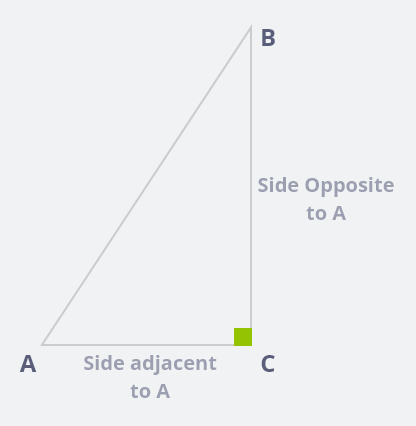Consider the above mentioned triangle as it the right-angle triangle. Here, the side opposite to angle consideration is known as perpendicular. The side adjacent to angle under consideration is known as base. The side opposite to right angle is known as hypotenuse.
A squared plus B squared equal to C squared, according to Pythagoras Theorem, the sum of the square of two sides i-e; perpendicular and base is equal to the square of the hypotenuse.

Hence, According to above mentioned figure.

$$\bar{AC} = Base$$

$$\bar{BC} = Perpendicular$$

$$\bar{AB} = Hypotenuse$$

So $$(Hyp)^2 = (Perpendicular)^2 + (Base)^2$$

$$(\bar{AB})^2 = (\bar{BC})^2 + (\bar{AC})^2$$

$$c^2 = a^2 + b^2$$

## Why do we use Pythagoras Formula?

Whenever we are facing any scenario where right angled triangle to involved and we are interested to find out any unknown sides by using two shared side, we establish a relation among all three easily by applying Pythagoras Theorem.

The Pythagoras Theorem consists of a formula $$(Hyp)^2 = (Perpendicular)^2 + (Base)^2$$ which is used to figure out the value of unknown side. We can use Pythagoras theorem in trigonometry ratios, measurement of distance, height and slant distance.

## Proof solver (solving for “a” or “b”) by Pythagoras theorem

Step no 1: consider the figure or object
Step no 2: Substitute the value in the Pythagoras theorem
Step no 3: Simplify the equation; make subject of the equation of unknown side.
Step no 4: take the square root

Example:

According to given diagram base ($$\bar{AC}$$) is unknown where a and c are shared sides: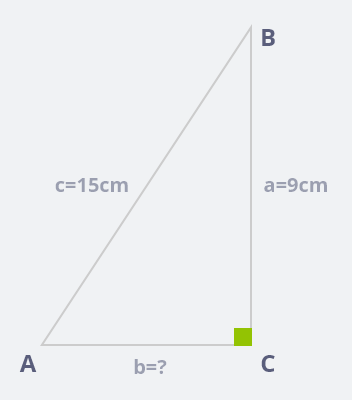Subsitute the given sides measurement in pythagoras theorem

$$(Hyp)^2 = (Perpendicular)^2 + (Base)^2$$

$$(\bar{AB})^2 = (\bar{BC})^2 + (\bar{AC})^2$$

$$(c)^2 = (a)^2 + (b)^2$$

Make unknown to subjectof the equation

$$(b)^2 = (c)^2 – (a)^2 = (15)^2 – (9)^2 = 225 – 81 = 144$$

Taking square root on both the sides

$$\sqrt{b^2} = \sqrt{144} = 12cm$$

## Application of Pythagoras theorem

1- It is useful for the two dimensional navigation.

2- To calculate the slope of the roof.

3- To find the length and height

4- To find the steepness of the mountain and temples.

5- To construct proper drainage system.

6- To construct dam and bridges.

7- To sailing and space light.

8- In baseball

9- To surveying the land

10- To find the shortest travel route.

Example 1: How heigh does a boy on the inclined plane?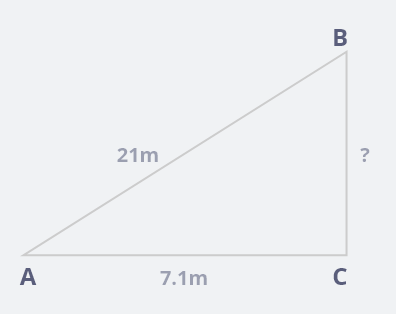Here,

$$\bar{AB} = 21m$$

$$\bar{AC} = 7.1m$$

$$\bar{BC} = ?$$

According to Pythagoras Theorem: $$(Hyp)^2 = (Perpendicular)^2 + (Base)^2$$

$$c^2 = a^2 + b^2$$

$$(21)^2 = a^2 + (7.1)^2$$

$$a^2 = (21)^2 – (7.1)^2$$

$$a^2 = 441 – 50.41$$

$$a^2 = 390.59$$

Taking square root on both the sides

$$\sqrt{a^2} = \sqrt{390.59}$$

$$a = 19.76m$$

Example 2: A 30 meter ladder is learning against the wall such that the base of the ladder is 7m from the base of the building. How far above ground is the touching point, where the ladder touches the building?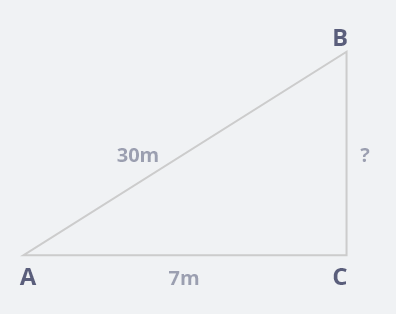Here,

$$\bar{AB} = 30m$$

$$\bar{AC} = 7m$$

$$\bar{BC} = ?$$

According to Pythagoras Theorem: $$(Hyp)^2 = (Perpendicular)^2 + (Base)^2$$

$$c^2 = a^2 + b^2$$

$$(30)^2 = a^2 + (7)^2$$

$$a^2 = (30)^2 – (7)^2$$

$$a^2 = 900 – 49$$

$$a^2 = 851$$

Taking square root on both the sides

$$\sqrt{a^2} = \sqrt{851}$$

$$a = 29.17m$$

Hence; the required touching point of the ladder is 29.17m.

Example 3: A rectangle is 8m wide and 14m long how long is the diagonal of the rectangle?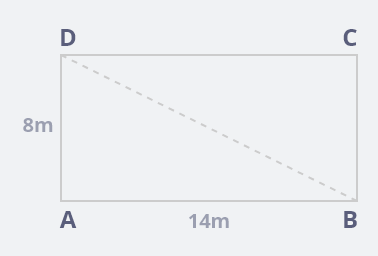Here,

Width is 8m

Length is 14m

The length of the diagonal = ?

By considering triangle △DAB, where b = 8m and d = 14m and x = ?

As it is the right angled triangle, we can easily apply pythagoras theorem

Therefore,

$$x^2 = a^2 + b^2$$

$$(x)^2 = (8)^2 + (14)^2$$

$$x^2 = 64 – 196$$

$$x^2 = 260m^2$$

Taking square root on both the sides

$$\sqrt{x^2} = \sqrt{260}$$

$$x = 16.12m$$

Hence, the length of the diagonal is 16.12m.

Example 4: Sara drove her car due east for 10 miles she then turned south and drove 30 miles. How far was she from she started her journey?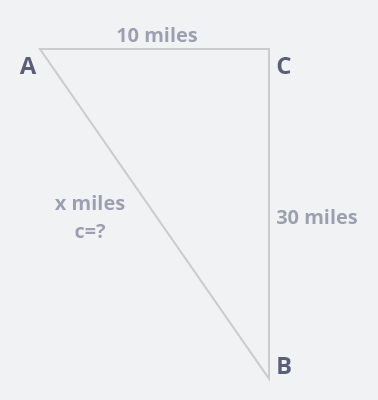Here,

a = 30 miles

b = 10 miles

c = ?

According to Pythagoras Theorem: $$(Hyp)^2 = (Perpendicular)^2 + (Base)^2$$

As: $$\bar{AB} = c = x$$ miles is unknown and according to given scenario, it is the hypotenuse. So,

$$c^2 = a^2 + b^2$$

$$(x)^2 = (30)^2 + (10)^2$$

$$(x)^2 = 900 + 100$$

$$x^2 = 1000$$

Taking square root on both the sides

$$\sqrt{x^2} = \sqrt{1000}$$

$$x = 31.62 miles$$

She is 31.62 miles away from the started point.

Example 5: Find the height of an equilateral triangle whose sides are 7cm long.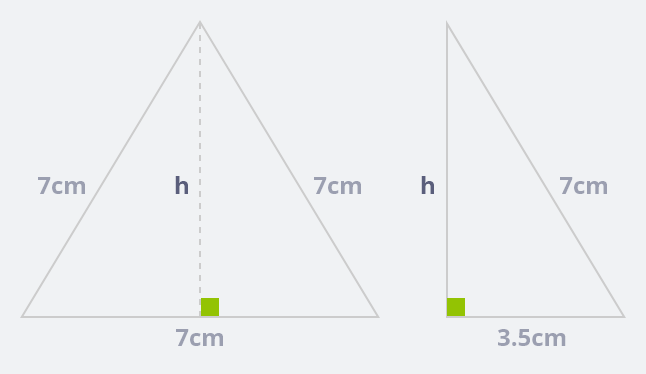According to Pythagoras Theorem: $$(Hyp)^2 = (Perpendicular)^2 + (Base)^2$$

$$(7)^2 = (h)^2 + (3.5)^2$$

$$49 = h^2 + 12.25$$

$$h^2 = 49 – 12.25$$

$$h^2 = 36.75$$

Taking square root on both the sides

$$\sqrt{h^2} = \sqrt{36.75}$$

$$h = 6.062$$

## Identify which of the following are Pythagorean Triplet

### 1- (3, 5, 4)

Solution:

$$3^2 + 4^2 = 9 + 16 = 25$$ and $$5^2 = 25$$

Since, the sum of the square of smaller numbers is equal to the square of the largest number.

So, (3, 4, 5) is the Pythagorean Triplet.

### 2- (4, 9, 12)

Solution:

$$4^2 + 9^2 = 16 + 81 = 97$$ and $$12^2 = 144$$

So, (4, 9, 12) is not a Pythagorean Triplet.

## What does squared mean? What is 13 squared?

The square of a number is the equivalent of a number multiplied by itself.

For example: The square of 3 is 9, because 3 ✕ 3 = 9. This is denoted by 3^2 = 9.

In mathematics, the square symbol (▢)2 is an arithmetic operation that signifies multiplying a number. The square of a number is the product of itself. Multiply a number by itself is called “squaring”, the number squaring a number is the same as raising that number to the power of two.

Therefore; In short; we can conclude the meaning of squaring in simple wording; the “square” means to calculate the value of a number multiplied by itself.

Hence; 13 squared is: 13 ✕ 13 = 169 means 13^2 = 169

## What does $$a^2 + b^2 = c^2$$ mean?

$$a^2 + b^2 = c^2$$ is known as the Pythagoras equation, named after the ancient Greek mathematician Pythagoras. This relationship is useful, if two sides of a right angle triangle is given and third one is required, the Pythagorean Theorem can be used to determine the length of the third side. In equation $$a^2 + b^2 = c^2$$ is the hypotenuse, “b” is the adjacent side and “a” is the side opposite to angle A.This equation reflect the meaningful picture of right angle triangle, the hypotenuse is equal to the sum of the square of the remaining two sides.

## FAQS

Q1: What means of Pythagoras?

Answer: Pythagoras theorem explains the relationship between base, perpendicular and hypotenuse of a right angled triangle.

Q2: What does $$a^2 + b^2 = c^2$$ reflect?

Answer: Here a, b and c are the sides of right angle triangle, in technical term c is the hypotenuse, b is the adjacent side and a is the side opposite to angle A. the square of the hypotenuse is equal to the sum of the squares of the remaining two sides.

Q3: Does Pythagoras theorem applicable on any triangle?

Answer: No, it is not applicable on all triangles except right angle triangle. So we can only apply on right angle triangle.

Q4: Is △ABC a right angles triangle, where AB = 6cm, BC = 11cm and AC = 16cm?

Answer: For filling the condition, it should satisfy the Pythagoras theorem.

$$(\bar{AB})^2 + (\bar{BC})^2 = (\bar{AC})^2$$

$$(6)^2 + (11)^2 = 157$$

And $$(\bar{AC})^2 = (16)^2 = 256$$

Hence, △ABC is not a right angles triangle.

Q5: What will be the distance of the foot of the ladder from the wall, if the length of the ladder is 10cm and height of the wall where ladder touch is 5cm

According to Pythagoras Theorem: $$(Hyp)^2 = (Perpendicular)^2 + (Base)^2$$

$$(10)^2 = (5)^2 + (Base)^2$$

$$(Base)^2 = (10)^2 – (5)^2$$

$$(Base)^2 = 100 – 25$$

$$(Base)^2 = 75 = 5\sqrt{3}$$

Hence; the distance between the ladder and wall is $$5\sqrt{3}$$

Q6: Which one is the important property of right angle triangle?

Answer: Pythagoras theorem is known as the important property.

Q7: Why $$a^2 + b^2 = c^2$$ is called Pythagoras theorem?

Answer: Because it was first discovered by Pythagoras (a Greek scientist)

Q8: How do we find a hypotenuse?

Answer: The hypotenuse is termed as the longest side of the right-angled triangle, for finding the longest side consider the relation /(a^2 + b^2 = c^2/), where $$c^2$$ is the sum of the square; which one is the longest-side (hypotenuse).

Q9: Where is Pythagoras theorem used?

– It is used for two-dimensional navigation

– To calculate the slop of the roof

– To find the steepness of the temples mountain

– To surveying the land

– To find the shortest travel route

Q10: What are the legs of the triangle?

Answer: Normally a and b known as legs of the triangle when angle c is considered right angle triangle.

Q11: What type of triangle, we can consider in Pythagoras theorem?

Answer: The Pythagoras theorem is true for right angle triangle only.

Q12: What do you understand by Pythagorean triplet?

Answer: The sum of the square of the smaller numbers is equal to the square of the largest number.

Q13: How can we identify the Pythagorean triplet?

Answer: By squaring the smaller numbers and equating the answer with the largest number square

Q14: Is side C on a triangle always the hypotenuse?

Answer: No, it depend on the vertices shared name, for example in below triangles.
In first triangle C is not the hypotenuse but in second figure C is considered hypotenuse as it the opposite of right-angled triangle.Q15: Which side of a right angled triangle is always the longest?

Answer: The hypotenuse is the longest side in a right angle triangle, as it is the opposite of the largest angle (i-e, 90°) as remaining two angles are less than 90°.

Q16: How do you know, three sides make a right-triangle?

Answer: If the smaller two sides squared is equal to the longest given side squared; then we can say that three sides can make a right angle triangle.

Q17: How old is Pythagoras theorem?

Answer: The Pythagoras theorem was first known in ancient Babylon and Egypt. The relationship was old as 400 year, according to ideal estimation its beginning is about 1900 B.C.

• Embed Calculator Widget
• Direct URL